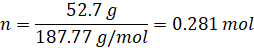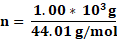It looks like you're using Internet Explorer 11 or older. This website works best with modern browsers such as the latest versions of Chrome, Firefox, Safari, and Edge. If you continue with this browser, you may see unexpected results.

# Chemistry

Stoichiometry

In a chemical reaction, stoichiometry is the quantitative description of the proportions of products and reactants. In order to use stoichiometry to determine the quantitative data of either reactants or products, one should be able to balance chemical reactions first.

Whether you're aiming to calculate the number of moles, volume, or mass using stochiometry, you can use the following order of steps in your calculation:

1) Write a balanced chemical equation.

2) Calculate the amount of starting specie using the information given to you in moles. You can use the following equations to find the moles of starting material depending on its type:

Solids

n = m/M

Solutions

n = CV

Gases

n = PV/RT

3) Determine if there is a limiting reagent.

4) Calculate the number of moles of desired specie by using molar ratio.

5) With the number of moles calculated, you can then calculate the desired quantity using the same formula as step 2 above.

Example 1

How many moles of Na2S2O3 is needed to react completely with 52.7 g of AgBr?

Na2S2O3 + AgBr   NaBr + Na3[Ag(S2O3)2]

Step 1: Balance the chemical equation:

2Na2S2O3 + AgBr  NaBr + Na3[Ag(S2O3)2]

Step 2: Calculate the amount of starting specie (AgBr) in moles using the following formula:

n = m/M

m (mass) = 52.7g

M (molar mass) = (107.87 g/mol) + (79.90 g/mol) = 187.77 g/molStep 3: Determine that the limiting reagent is AgBr, as it is in a limited supply of 52.7g (0.281 mol)

Step 4: Calculate the desired amount of Na2S2O3 needed to react with 0.281 mols of AgBr using the molar ratio from the balanced equation.Solving for "X" in the above equation gives:

X = 0.562 mol

Therefore, the amount of Na2S2O3  needed is 0.562 mol to react with 0.281 mol of AgBr.

Example 2

Carbon dioxide reacts with lithium hydroxide to produce lithium carbonate and water. What mass of lithium hydroxide is required to react with 1.00 x103 grams of carbon dioxide?

Step 1: Write the balanced equation as shown below:

CO2(g) + 2LiOH(s)     Li2CO3(s) + H2O(g)

Step 2: Calculate the amount of starting specie (CO2) in moles using the following formula:

n = m/M

m (mass) = 2.00 x103g

M (molar mass) = (12.01 g/mol) + (16.00 g/mol) = 44.01 g/mol= 22.7 mols

Step 3: Determine the limiting reagent is CO2 as it is in a limited supply of 1.00 x103g (22.7 mols)

Step 4: Calculate the desired amount of LiOH needed to react with 22.7 mols of CO2 using the molar ratio from the balanced equation.Solving for "X" in the above equation gives:

X = 45.4 mol

Therefore, the amount of LiOH needed is 45.4 mol to react with 22.7 mol of AgBr.

From the mols of LiOH calculated, the mass can be calculated using the following formula:

n = m/M

m (mass) = ?

M (molar mass) = (6.94 g/mol) + (16.00 g/mol) +(1.01 g/mol) = 23.95 g/mol

m = n*M = 22.7 mol * 23.95 g/mol = 543.665 g = 5.44 x102g